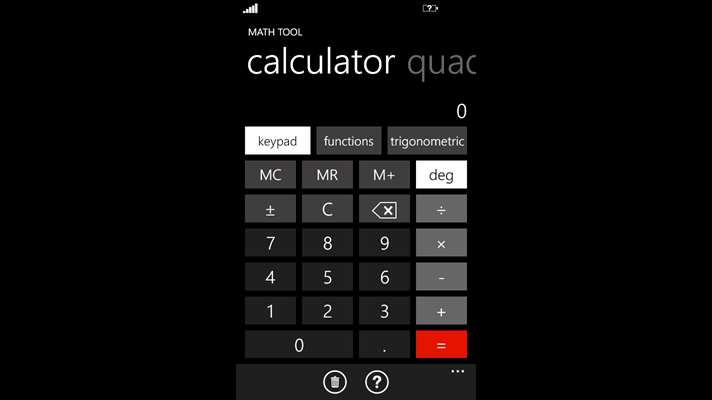This app is the most Advanced Math Tool in the Windows Phone Store which includes Includes many powerful tools of great value for students, teachers, engineers and many more, all in a great, stylish package.

## Math Tool Features:

• Scientific Calculator (36+ useful math functions) • Quadratic Equation solver • Linear Interpolation tool • Logarithmic Interpolation tool • Linear Least Squares Regression • Quadratic Least Squares Regression